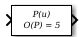Documentation

### This is machine translation

Mouseover text to see original. Click the button below to return to the English version of the page.

To view all translated materials including this page, select Country from the country navigator on the bottom of this page.

# Polynomial

Perform evaluation of polynomial coefficients on input values

• Library:
• Simulink / Math Operations## Description

The Polynomial block evaluates `P(u)` at each time step for the input u. You define a set of polynomial coefficients in the form that the MATLAB® `polyval` command accepts.

## Ports

### Input

expand all

Value at which to evaluate the polynomial P(u).

Data Types: `single` | `double`

### Output

expand all

Value of the polynomial P(u) evaluated at the input signal.

Data Types: `single` | `double`

## Parameters

expand all

Specify polynomial coefficients in MATLAB `polyval` form. The first coefficient corresponds to xN and the remaining coefficients correspond to decreasing orders of x. The last coefficient represents the constant for the polynomial.

#### Programmatic Use

 Block Parameter: `coefs` Type: real array Default: ```[ +2.081618890e-019, -1.441693666e-014, +4.719686976e-010, -8.536869453e-006, +1.621573104e-001, -8.087801117e+001 ]```

## Block Characteristics

 Data Types `double` | `single` Direct Feedthrough `yes` Multidimensional Signals `no` Variable-Size Signals `no` Zero-Crossing Detection `no`